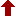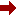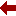#### SLIM21

 Sort Order Chronological Author Title ItemType,Author ItemType,Title Display Format Tabular Publication Bibliography Items / Page 5 10 25

Click the serial number on the left to view the details of the item.
#  AuthorTitleAccn#YearItem Type Claims
1 Kantorovich, Lev Mathematics for Natural Scientists I12528 2022 Book
2 Milstein, Grigori N Stochastic Numerics for Mathematical Physics I11924 2021 eBook
3 Saravanan, Rajendran Solvable One-Dimensional Multi-State Models for Statistical and Quantum Mechanics I11908 2021 eBook
4 Selinger, Jonathan V Introduction to the Theory of Soft Matter I10283 2016 eBook
5 Kantorovich, Lev Mathematics for Natural Scientists II I10062 2016 eBook
6 Sahni, Viraht Quantal Density Functional Theory I09642 2016 eBook
7 Kantorovich, Lev Mathematics for Natural Scientists I09133 2016 eBook
8 Conte, Robert The Painlev?? Handbook I09128 2020 eBook
9 Seaborn, James B Mathematics for the Physical Sciences I11155 2002 eBook
10 Hassani, Sadri Mathematical Methods Using Mathematica?? I10927 2003 eBook
 (page:1 / 4) [#35] Next Page Last Page

1.TitleMathematics for Natural Scientists : Fundamentals and Basics
Author(s)Kantorovich, Lev
PublicationCham, 1. Imprint: Springer 2. Springer International Publishing, 2022.
DescriptionXXIII, 768 p. 189 illus., 163 illus. in color : online resource
Abstract NoteThis book, now in a second revised and enlarged edition, covers a course of mathematics designed primarily for physics and engineering students. It includes all the essential material on mathematical methods, presented in a form accessible to physics students and avoiding unnecessary mathematical jargon and proofs that are comprehensible only to mathematicians. Instead, all proofs are given in a form that is clear and sufficiently convincing for a physicist. Examples, where appropriate, are given from physics contexts. Both solved and unsolved problems are provided in each section of the book. The second edition includes more on advanced algebra, polynomials and algebraic equations in significantly extended first two chapters on elementary mathematics, numerical and functional series and ordinary differential equations. Improvements have been made in all other chapters, with inclusion of additional material, to make the presentation clearer, more rigorous and coherent, and the number of problems has been increased at least twofold. Mathematics for Natural Scientists: Fundamentals and Basics is the first of two volumes. Advanced topics and their applications in physics are covered in the second volume the second edition of which the author is currently being working on
ISBN,Price9783030912222
Keyword(s)1. Applications of Mathematics 2. Chemometrics 3. Diseases 4. EBOOK 5. EBOOK - SPRINGER 6. ENGINEERING MATHEMATICS 7. Engineering???Data processing 8. Mathematical and Computational Engineering Applications 9. Mathematical Applications in Chemistry 10. Mathematical Methods in Physics 11. MATHEMATICAL PHYSICS 12. MATHEMATICS 13. Theoretical, Mathematical and Computational Physics
Item TypeBook
Circulation Data
 Accession# Call# Status Issued To Return Due On Physical Location I12528 On Shelf

2.TitleStochastic Numerics for Mathematical Physics
Author(s)Milstein, Grigori N;Tretyakov, Michael V
PublicationCham, Springer International Publishing, 2021.
DescriptionXXV, 736 p. 33 illus : online resource
Abstract NoteThis book is a substantially revised and expanded edition reflecting major developments in stochastic numerics since the first edition was published in 2004. The new topics, in particular, include mean-square and weak approximations in the case of nonglobally Lipschitz coefficients of Stochastic Differential Equations (SDEs) including the concept of rejecting trajectories; conditional probabilistic representations and their application to practical variance reduction using regression methods; multi-level Monte Carlo method; computing ergodic limits and additional classes of geometric integrators used in molecular dynamics; numerical methods for FBSDEs; approximation of parabolic SPDEs and nonlinear filtering problem based on the method of characteristics. SDEs have many applications in the natural sciences and in finance. Besides, the employment of probabilistic representations together with the Monte Carlo technique allows us to reduce the solution of multi-dimensional problems for partial differential equations to the integration of stochastic equations. This approach leads to powerful computational mathematics that is presented in the treatise. Many special schemes for SDEs are presented. In the second part of the book numerical methods for solving complicated problems for partial differential equations occurring in practical applications, both linear and nonlinear, are constructed. All the methods are presented with proofs and hence founded on rigorous reasoning, thus giving the book textbook potential. An overwhelming majority of the methods are accompanied by the corresponding numerical algorithms which are ready for implementation in practice. The book addresses researchers and graduate students in numerical analysis, applied probability, physics, chemistry, and engineering as well as mathematical biology and financial mathematics
ISBN,Price9783030820404
Keyword(s)1. BIOMATHEMATICS 2. Chemometrics 3. Computational Science and Engineering 4. EBOOK 5. EBOOK - SPRINGER 6. ENGINEERING MATHEMATICS 7. Engineering???Data processing 8. Mathematical and Computational Biology 9. Mathematical and Computational Engineering Applications 10. Mathematical Applications in Chemistry 11. MATHEMATICAL PHYSICS 12. Mathematics in Business, Economics and Finance 13. Mathematics???Data processing 14. Social sciences???Mathematics 15. Theoretical, Mathematical and Computational Physics
Item TypeeBook
Circulation Data
 Accession# Call# Status Issued To Return Due On Physical Location I11924 On Shelf

3.TitleSolvable One-Dimensional Multi-State Models for Statistical and Quantum Mechanics
Author(s)Saravanan, Rajendran;Chakraborty, Aniruddha
PublicationSingapore, Springer Nature Singapore, 2021.
DescriptionXIX, 174 p. 94 illus., 44 illus. in color : online resource
Abstract NoteThis book highlights the need for studying multi-state models analytically for understanding the physics of molecular processes. An intuitive picture about recently solved models of statistical and quantum mechanics is drawn along with presenting the methods developed to solve them. The models are relevant in the context of molecular processes taking place in gaseous phases and condensed phases, emphasized in the introduction. Chapter 1 derives the arisal of multi-state models for molecular processes from the full Hamiltonian description. The model equations are introduced and the literature review presented in short. In Chapter 2, the time-domain methods to solve Smoluchowski-based reaction-diffusion systems with single-state and two-state descriptions are discussed. Their corresponding analytical results derive new equilibrium concepts in reversible reactions and studies the effect of system and molecular parameters in condensed-phase chemical dynamics. In Chapter 3, time-domain methods to solve quantum scattering problems are developed. Along side introducing a brand new solvable model in quantum scattering, it discusses transient features of quantum two-state models. In interest with electronic transitions, a new solvable two-state model with localized non-adiabatic coupling is also presented. The book concludes by proposing the future scope of the model, thereby inviting new research in this fundamentally important and rich applicable field
ISBN,Price9789811666544
Keyword(s)1. Chemometrics 2. EBOOK 3. EBOOK - SPRINGER 4. Mathematical Applications in Chemistry 5. MATHEMATICAL PHYSICS 6. MATHEMATICAL STATISTICS 7. Theoretical, Mathematical and Computational Physics
Item TypeeBook
Circulation Data
 Accession# Call# Status Issued To Return Due On Physical Location I11908 On Shelf

4.TitleIntroduction to the Theory of Soft Matter : From Ideal Gases to Liquid Crystals
Author(s)Selinger, Jonathan V
PublicationCham, Springer International Publishing, 2016.
DescriptionX, 185 p. 78 illus., 69 illus. in color : online resource
Abstract NoteThis book presents the theory of soft matter to students at the advanced undergraduate or beginning graduate level. It provides a basic introduction to theoretical physics as applied to soft matter, explaining the concepts of symmetry, broken symmetry, and order parameters; phases and phase transitions; mean-field theory; and the mathematics of variational calculus and tensors. It is written in an informal, conversational style, which is accessible to students from a diverse range of backgrounds. The book begins with a simple ???toy model??? to demonstrate the physical significance of free energy. It then introduces two standard theories of phase transitions???the Ising model for ferromagnetism and van der Waals theory of gases and liquids???and uses them to illustrate principles of statistical mechanics. From those examples, it moves on to discuss order, disorder, and broken symmetry in many states of matter, and to explain the theoretical methods that are used to model the phenomena. It concludes with a chapter on liquid crystals, which brings together all of these physical and mathematical concepts. The book is accompanied by a set of ???interactive figures,??? which allow online readers to change parameters and see what happens to a graph, some allow users to rotate a plot or other graphics in 3D, and some do both. These interactive figures help students to develop their intuition for the physical meaning of equations. This book will prepare advanced undergraduate or early graduate students to go into more advanced theoretical studies. It will also equip students going into experimental soft matter science to be fully conversant with the theoretical aspects and have effective collaborations with theorists
ISBN,Price9783319210544
Keyword(s)1. Amorphous substances 2. Biomedical engineering 3. Biomedical Engineering and Bioengineering 4. Chemometrics 5. Complex fluids 6. EBOOK 7. EBOOK - SPRINGER 8. Math. Applications in Chemistry 9. PHYSICAL CHEMISTRY 10. Soft and Granular Matter, Complex Fluids and Microfluidics
Item TypeeBook
Circulation Data
 Accession# Call# Status Issued To Return Due On Physical Location I10283 On Shelf

5.TitleMathematics for Natural Scientists II : Advanced Methods
Author(s)Kantorovich, Lev
PublicationCham, Springer International Publishing, 2016.
DescriptionXVII, 675 p. 107 illus., 104 illus. in color : online resource
Abstract NoteThis book covers the advanced mathematical techniques useful for physics and engineering students, presented in a form accessible to physics students, avoiding precise mathematical jargon and laborious proofs. Instead, all proofs are given in a simplified form that is clear and convincing for a physicist. Examples, where appropriate, are given from physics contexts. Both solved and unsolved problems are provided in each chapter. Mathematics for Natural Scientists II: Advanced Methods is the second of two volumes. It follows the first volume on Fundamentals and Basics
ISBN,Price9783319278612
Keyword(s)1. APPLIED MATHEMATICS 2. Chemometrics 3. EBOOK 4. EBOOK - SPRINGER 5. ENGINEERING MATHEMATICS 6. Math. Applications in Chemistry 7. Mathematical and Computational Engineering 8. Mathematical Applications in the Physical Sciences 9. Mathematical Methods in Physics 10. MATHEMATICAL PHYSICS 11. Numerical and Computational Physics, Simulation 12. PHYSICS
Item TypeeBook
Circulation Data
 Accession# Call# Status Issued To Return Due On Physical Location I10062 On Shelf

6.TitleQuantal Density Functional Theory
Author(s)Sahni, Viraht
PublicationBerlin, Heidelberg, Springer Berlin Heidelberg, 2016.
DescriptionXIX, 413 p. 71 illus., 20 illus. in color : online resource
Abstract NoteThis book deals with quantal density functional theory (QDFT) which is a time-dependent local effective potential theory of the electronic structure of matter. The treated time-independent QDFT constitutes a special case. In the 2nd edition, the theory is extended to include the presence of external magnetostatic fields. The theory is a description of matter based on the ???quantal Newtonian??? first and second laws which is in terms of ???classical??? fields that pervade all space, and their quantal sources. The fields, which are explicitly defined, are separately representative of electron correlations due to the Pauli exclusion principle, Coulomb repulsion, correlation-kinetic, correlation-current-density, and correlation-magnetic effects. The book further describes Schr??dinger theory from the new physical perspective of fields and quantal sources. It also describes traditional Hohenberg-Kohn-Sham DFT, and explains via QDFT the physics underlying the various energy functionals and functional derivatives of the traditional approach to electronic structure.
ISBN,Price9783662498422
Keyword(s)1. Chemometrics 2. EBOOK 3. EBOOK - SPRINGER 4. Interfaces (Physical sciences) 5. Materials???Surfaces 6. Math. Applications in Chemistry 7. MATHEMATICAL PHYSICS 8. Surface and Interface Science, Thin Films 9. Surfaces (Physics) 10. Surfaces and Interfaces, Thin Films 11. Theoretical, Mathematical and Computational Physics 12. THIN FILMS
Item TypeeBook
Circulation Data
 Accession# Call# Status Issued To Return Due On Physical Location I09642 On Shelf

7.TitleMathematics for Natural Scientists : Fundamentals and Basics
Author(s)Kantorovich, Lev
PublicationNew York, NY, Springer New York, 2016.
DescriptionXVII, 526 p. 124 illus., 118 illus. in color : online resource
Abstract NoteThis book, the first in a two part series, covers a course of mathematics tailored specifically for physics, engineering and chemistry students at the undergraduate level. It is unique in that it begins with logical concepts of mathematics first encountered at A-level and covers them in thorough detail, filling in the gaps in students' knowledge and reasoning. Then the book aids the leap between A-level and university-level mathematics, with complete proofs provided throughout and all complex mathematical concepts and techniques presented in a clear and transparent manner. Numerous examples and problems (with answers) are given for each section and, where appropriate, mathematical concepts are illustrated in a physics context. This text gives an invaluable foundation to students and a comprehensive aid to lecturers. Mathematics for Natural Scientists: Fundamentals and Basics is the first of two volumes. Advanced topics and their applications in physics are covered in the second volume
ISBN,Price9781493927852
Keyword(s)1. APPLIED MATHEMATICS 2. Chemometrics 3. EBOOK 4. EBOOK - SPRINGER 5. ENGINEERING MATHEMATICS 6. Math. Applications in Chemistry 7. Mathematical and Computational Engineering 8. Mathematical Applications in the Physical Sciences 9. Mathematical Methods in Physics 10. MATHEMATICAL PHYSICS 11. Numerical and Computational Physics, Simulation 12. PHYSICS
Item TypeeBook
Circulation Data
 Accession# Call# Status Issued To Return Due On Physical Location I09133 On Shelf

8.TitleThe Painlev?? Handbook
Author(s)Conte, Robert;Musette, Micheline
PublicationCham, Springer International Publishing, 2020.
DescriptionXXXI, 389 p. 15 illus., 6 illus. in color : online resource
Abstract NoteThis book, now in its second edition, introduces the singularity analysis of differential and difference equations via the Painlev?? test and shows how Painlev?? analysis provides a powerful algorithmic approach to building explicit solutions to nonlinear ordinary and partial differential equations. It is illustrated with integrable equations such as the nonlinear Schr??dinger equation, the Korteweg-de Vries equation, H??non-Heiles type Hamiltonians, and numerous physically relevant examples such as the Kuramoto-Sivashinsky equation, the Kolmogorov-Petrovski-Piskunov equation, and mainly the cubic and quintic Ginzburg-Landau equations. Extensively revised, updated, and expanded, this new edition includes: recent insights from Nevanlinna theory and analysis on both the cubic and quintic Ginzburg-Landau equations; a close look at physical problems involving the sixth Painlev?? function; and an overview of new results since the book???s original publication with special focus on finite difference equations. The book features tutorials, appendices, and comprehensive references, and will appeal to graduate students and researchers in both mathematics and the physical sciences
ISBN,Price9783030533403
Keyword(s)1. APPLIED MATHEMATICS 2. Chemometrics 3. Dynamical Systems and Ergodic Theory 4. DYNAMICS 5. EBOOK 6. EBOOK - SPRINGER 7. ENGINEERING MATHEMATICS 8. ERGODIC THEORY 9. Math. Applications in Chemistry 10. Mathematical and Computational Engineering 11. Mathematical Methods in Physics 12. MATHEMATICAL PHYSICS 13. PARTIAL DIFFERENTIAL EQUATIONS 14. PHYSICS
Item TypeeBook
Circulation Data
 Accession# Call# Status Issued To Return Due On Physical Location I09128 On Shelf

9.TitleMathematics for the Physical Sciences
Author(s)Seaborn, James B
PublicationNew York, NY, Springer New York, 2002.
DescriptionXI, 245 p : online resource
Abstract NoteThis book is intended to provide a mathematical bridge from a general physics course to intermediate-level courses in classical mechanics, electricity and mag?? netism, and quantum mechanics. The book begins with a short review of a few topics that should be familiar to the student from a general physics course. These examples will be used throughout the rest of the book to provide physical con?? texts for introducing the mathematical applications. The next two chapters are devoted to making the student familiar with vector operations in algebra and cal?? culus. Students will have already become acquainted with vectors in the general physics course. The notion of magnetic flux provides a physical connection with the integral theorems of vector calculus. A very short chapter on complex num?? bers is sufficient to supply the needed background for the minor role played by complex numbers in the remainder of the text. Mathematical applications in in?? termediate and advanced undergraduate courses in physics are often in the form of ordinary or partial differential equations. Ordinary differential equations are introduced in Chapter 5. The ubiquitous simple harmonic oscillator is used to il?? lustrate the series method of solving an ordinary, linear, second-order differential equation. The one-dimensional, time-dependent SchrOdinger equation provides an illus?? tration for solving a partial differential equation by the method of separation of variables in Chapter 6
ISBN,Price9781468492798
Keyword(s)1. APPLIED MATHEMATICS 2. Chemometrics 3. EBOOK 4. EBOOK - SPRINGER 5. ENGINEERING MATHEMATICS 6. Math. Applications in Chemistry 7. Mathematical and Computational Engineering 8. Mathematical Methods in Physics 9. Numerical and Computational Physics, Simulation 10. PHYSICS
Item TypeeBook
Circulation Data
 Accession# Call# Status Issued To Return Due On Physical Location I11155 On Shelf

10.TitleMathematical Methods Using Mathematica?? : For Students of Physics and Related Fields